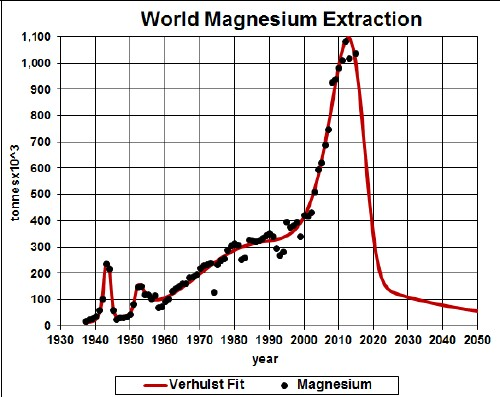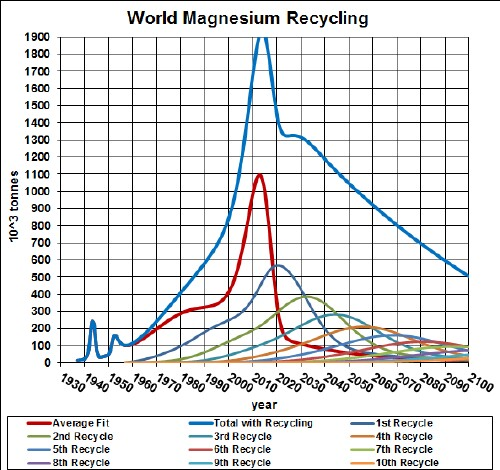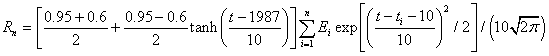# Magnesium Depletion and Recycling

Magnesium is used in batteries, paints and plastics. The graph below shows the magnesium extraction data for the world and a Verhulst function fit to the data in order to extrapolate into the future.Magnesium extraction rate for the world and two Verhulst function fits to the data.

Since there is a large reserves for magnesium, there will probably be one or more future peaks for magnesium extraction. Magnesium can also be obtained from sea water.

## Recycling

Assume that:

• Recycling of magnesium follows a hyperbolic tangent curve from 60% to 95% recycling with a break point of year 1987 and width 10 years.
• The recycling is delayed by a Gaussian curve peaking at a delay of 10 years and a width of 10 years.

The effective magnesium available for making items after the first ten recycling cycles is shown in the following graph, along with the effective magnesium available for each cycle:The equation for a recyling cycle is,

where Ei is the amount available from the previous cycle. Here is an example of the Excel coding:

{=((\$J\$2+\$I\$2)/2+((\$J\$2-\$I\$2)/2)*TANH((A27-\$K\$2)/\$L\$2))*SUM(\$I\$27:I27*(EXP(-1*((A27-\$A\$27:A27-\$N\$2)/\$O\$2)^2/2))/\$O\$2/SQRT(2*PI()))} (The curly bracket surrounding the term makes it into an array; it must be entered by holding down the SHIFT & CTRL keys while pressing the ENTER key.)

The Excel spreadsheet is set up to make it easy to calculate with different recycling assumptions.

Minerals Depletion